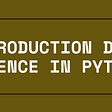# How do I apply the T-Test correctly?

Hi everyone,

I am not sure if I apply the T-Test correctly to my data. In the description it says “H = TTEST(X) performs a t-test of the hypothesis that the data in the vector X come from a distribution with mean zero, and returns the result of the test in H.” That is my code:

`load stockreturnsx = stocks(:,3);x1=x-mean(x);x2=x+10;[h,p,ci,stats] = ttest(x,0,'Alpha',0.01);[h1,p1,ci1,stats1] = ttest(x1,0,'Alpha',0.01);[h2,p2,ci2,stats2] = ttest(x2,0,'Alpha',0.01);`

The way I understand it, the T-Test checks if your data is t-distributed with a mean of 0. If I know that my data has a mean of e.g. 10, the T-Test gives really bad results, because the mean is not 0. If I still want to find out if there is a t-distribution, can I substract 10 from my data and apply the T-Test without making any serious error?

]

If I substract the mean of my data (line 3) the P value becomes 1 in every case. Why is that?

I assume that when I substract the mean, the criterion of 0 mean is met. But that doesn’t necessarily mean that I have a T-distribution, right? So if I substract the mean of my values and p becomes 1, I have a T-distribution?

I’m sorry for my confusing words. I hope you understand what I mean.

NOTE:-

Matlabsolutions.com provide latest MatLab Homework Help,MatLab Assignment Help for students, engineers and researchers in Multiple Branches like ECE, EEE, CSE, Mechanical, Civil with 100% output.Matlab Code for B.E, B.Tech,M.E,M.Tech, Ph.D. Scholars with 100% privacy guaranteed. Get MATLAB projects with source code for your learning and research.

The ttest function is correct. Remember to look at the p-value — the second output — to get the calculated probability. The first output is a flag indicating that the null hypothesis that the mean is equal to zero is accepted (0) or rejected (1).

The ttest function returns the probability (the second output) that the mean of the data is zero, so when you subtract the mean (as you did in ‘x1’), that probability is exactly 1 (within floating-point precision), because it is as certain as it is possible to express that the mean is not different from zero, and the null hypothesis is accepted.

Note that for ‘x2’, ‘h2’ is 1 and the p-value is vanishingly small, indicating that there is an infinitesimal probability (on the order of 1/10⁷⁹) that the mean of your data is equal to zero, and the null hypothesis is rejected.

‘But that doesn’t necessarily mean that I have a T-distribution, right?’

--

--

--

## More from Technical Source

Simple! That is me, a simple person. I am passionate about knowledge and reading. That’s why I have decided to write and share a bit of my life and thoughts to.

Love podcasts or audiobooks? Learn on the go with our new app.

## The way you version control your ML projects is wrong## Covid-19 infection in Italy and air pollution. Is there a correlation?## Even if I die trying: Learning Data Science## Topic Modeling with Spanish text: an introductory example in Python## Today we’re sharing the story of Conrad Buchanan, a devoted husband and father who died after…## How Duplicate Corrode Your Salesforce Data and What You Can Do About It## How to do Unsupervised Clustering with Keras## Simulation## Technical Source

Simple! That is me, a simple person. I am passionate about knowledge and reading. That’s why I have decided to write and share a bit of my life and thoughts to.

## Python equivalent to Matlab function ‘imfill’ for grayscale images## Introduction to Data Science in Python## Day 9- Python with Reeborg’s World